# Hill Cipher

Let’s learn how Hill Cipher works and everything you need to know about Hill Cipher with its implementation.

When you are sending a text message to a friend, you don’t want your message to be manipulated or misused by an intruder. In order to avoid this, we need to convert the plain text data to a ciphertext. Before getting into this conversion let us first know what a ciphertext is.

## Ciphertext

A ciphertext is a formatted text which is not understood by anyone. Hill cipher is one of the techniques to convert a plain text into ciphertext and vice versa. There are two parts in the Hill cipher – Encryption and Decryption.

## Encryption – Plain text to Cipher text

Encryption is converting plain text into ciphertext. The working is shown below:

Input :
1.Plain text that has to be converted into ciphertext.
2.A KEY to encrypt the plain text
Output: Ciphertext

We have a simple formula for encryption
C = KPmod26
C  is ciphertext, K is the key, P is the plain text vector.

The KEY is generally given in the problem statement. Here we are considering a 2×2 matrix. The plain text vector is represented as a column matrices that are considered one at a time. Since the key matrix is 2×2, we take each column matrix as 2×1. If the key matrix was 3×3, then each column matrix would be 3×1.

let us take an example where plain text is ‘exam‘ which has to be converted to ciphertext with key-value as now, form the column matrices into 2×1 configurations and covert the text into numeric data assigning values to each alphabet from 0 to 25.
a=0,b=1,c=2,d=3,………….,y=24,z=25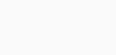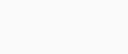Consider the first column matrix and substitute in the above formula: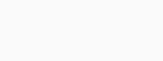repeat this for second column matrix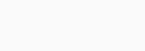Hence the final ciphertext is ‘elsc’

## Decryption – Cipher text to plain text

Decryption is the conversion of ciphertext into plain text. It can be done by a simple formula
P=(K’)(C) mod26
where P is the plain text, K’ is the inverse key matrix, C is the ciphertext vector or the column matrices.

Input: ciphertext and key
Output: plain text.

Here the C=’elsc’, which are further divided into column matrices:and K=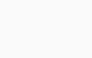Now let us see the working:

1. First, find the adjacent matrix of the given key matrix
K_adj=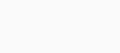2. Find the determinant of the key matrix
77-88=-11

3. Find the modulo of the determinant with 26
-11 mod26 =15=d

4. Find the inverse number of the above result
d x d’=1 mod26
15 x d’=1 mod26
d’=7

5. Any negative numbers in K_adj should be added by 26 and then the whole matrix is multiplied by d’.
K’ =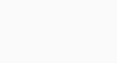Now, this is our new key matrix. Substituting all the values in the decryption formula, we get the required plain text.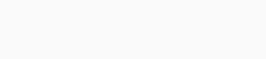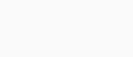Repeat the above step using the other column matrix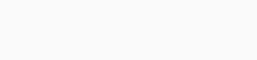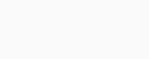Hence the final plain text is ‘exam’.

## Hill Cipher in Java

```import java.util.*;

import java.io.IOException;

public class HillCipherExample {
int[] l_m;
int[][] k_m;
int[] r_m;
static int ch;
int [][] nk;

public void perf_Division(string t, int str)
{
while (t.length() > str)
{
string l = t.substring(0, str);
t = t.substring(str, t.length());
calLineMatrix(l);
if(ch ==1){
multiplyLineByKey(l.length());
}else{
multiplyLineByInvKey(l.length());
}
showResult(l.length());
}
if (t.length() == str){

if(ch ==1){
calLineMatrix(t);
multiplyLineByKey(t.length());
showResult(t.length());
}
else{
calLineMatrix(t);
this.multiplyLineByInvKey(t.length());
showResult(t.length());
}
}
else if (t.length() < str)
{
for (int i = t.length(); i < str; i++)
t = t + 'x';
if(ch ==1){
calLineMatrix(t);
multiplyLineByKey(t.length());
showResult(t.length());
}
else{
calLineMatrix(t);
multiplyLineByInvKey(t.length());
showResult(t.length());
}
}
}

public void calKeyMatrix(string key, int len)
{
k_m = new int[len][len];
int k = 0;
for (int i = 0; i < len; i++)
{
for (int j = 0; j < len; j++)
{
k_m[i][j] = ((int) key.charAt(k)) - 97;
k++;
}
}
}

public void calLineMatrix(string l)
{
l_m = new int[l.length()];
for (int i = 0; i < l.length(); i++)
{
l_m[i] = ((int) l.charAt(i)) - 97;
}
}

public void multiplyLineByKey(int len)
{
r_m = new int[len];
for (int i = 0; i < len; i++)
{
for (int j = 0; j < len; j++)
{
r_m[i] += k_m[i][j] * l_m[j];
}
r_m[i] %= 26;
}
}
public void multiplyLineByInvKey(int len)
{

r_m = new int[len];
for (int i = 0; i < len; i++)
{
for (int j = 0; j < len; j++)
{
r_m[i] += nk[i][j] * l_m[j];
}
r_m[i] %= 26;
}
}

public void showResult(int len)
{
string result = "";
for (int i = 0; i < len; i++)
{
result += (char) (r_m[i] + 97);
}
system.out.print(result);
}

public int calDeter_minant(int A[][], int N)
{
int resultOfDet;
switch (N) {
case 1:
resultOfDet = A;
break;
case 2:
resultOfDet = A * A - A * A;
break;
default:
resultOfDet = 0;
for (int j1 = 0; j1 < N; j1++)
{
int m[][] = new int[N - 1][N - 1];
for (int i = 1; i < N; i++)
{
int j2 = 0;
for (int j = 0; j < N; j++)
{
if (j == j1)
continue;
m[i - 1][j2] = A[i][j];
j2++;
}
}
resultOfDet += Math.pow(-1.0, 1.0 + j1 + 1.0) * A[j1]
* calDeter_minant(m, N - 1);
}   break;
}
return resultOfDet;
}

public void cofact(int num[][], int f)
{
int b[][], fac[][];
b = new int[f][f];
fac = new int[f][f];
int p, q, m, n, i, j;
for (q = 0; q < f; q++)
{
for (p = 0; p < f; p++)
{
m = 0;
n = 0;
for (i = 0; i < f; i++)
{
for (j = 0; j < f; j++)
{
b[i][j] = 0;
if (i != q && j != p)
{
b[m][n] = num[i][j];
if (n < (f - 2))
n++;
else
{
n = 0;
m++;
}
}
}
}
fac[q][p] = (int) Math.pow(-1, q + p) * calDeter_minant(b, f - 1);
}
}
trans(fac, f);
}

void trans(int fac[][], int r)
{
int i, j;
int b[][], inv[][];
b = new int[r][r];
inv = new int[r][r];
int d = calDeter_minant(k_m, r);
int mi = mi(d % 26);
mi %= 26;
if (mi < 0)
mi += 26;
for (i = 0; i < r; i++)
{
for (j = 0; j < r; j++)
{
b[i][j] = fac[j][i];
}
}
for (i = 0; i < r; i++)
{
for (j = 0; j < r; j++)
{
inv[i][j] = b[i][j] % 26;
if (inv[i][j] < 0)
inv[i][j] += 26;
inv[i][j] *= mi;
inv[i][j] %= 26;
}
}

nk = inv;
}

public int mi(int d)
{
int q, r1, r2, r, t1, t2, t;
r1 = 26;
r2 = d;
t1 = 0;
t2 = 1;
while (r1 != 1 && r2 != 0)
{
q = r1 / r2;
r = r1 % r2;
t = t1 - (t2 * q);
r1 = r2;
r2 = r;
t1 = t2;
t2 = t;
}
return (t1 + t2);
}

public void matrixtoinvkey(int inv[][], int n)
{
string invkey = "";
for (int i = 0; i < n; i++)
{
for (int j = 0; j < n; j++)
{
invkey += (char) (inv[i][j] + 97);
}
}
system.out.print(invkey);
}
public boolean check(string key, int len)
{
calKeyMatrix(key, len);
int d = calDeter_minant(k_m, len);
d = d % 26;
if (d == 0)
{
system.out.println("Key is not invertible");
return false;
}
else if (d % 2 == 0 || d % 13 == 0)
{
system.out.println("Key is not invertible");
return false;
}
else
{
return true;
}
}

public static void main(string args[]) throws IOException
{
HillCipherExample obj = new HillCipherExample();
system.out.println("Enter the line: ");
string l = in.readLine();
system.out.println("Enter the key: ");
string key = in.readLine();
double sq = Math.sqrt(key.length());
if (sq != (long) sq)
system.out.println("Cannot For_m a square matrix");
else
{
int size = (int) sq;
if (obj.check(key, size))
{

system.out.println("Result:");
obj.cofact(obj.k_m, size);
obj.perf_Division(l, size);

}
}
}
}```
`Output:`## Hill Cipher in Python

```import numpy as np
def encryption(m):
# Replace spaces with nothing
m = m.replace(" ", "")
# Ask for keyword and get encryption matrix
C = make_key()
# Append zero if the messsage isn't divisble by 2
len_check = len(m) % 2 == 0
if not len_check:
m += "0"
# Populate message matrix
P = create_matrix_of_integers_from_string(m)
# Calculate length of the message
m_len = int(len(m) / 2)
# Calculate P * C
encrypted_m = ""
for i in range(m_len):
# Dot product
row_0 = P[i] * C + P[i] * C
# Modulate and add 65 to get back to the A-Z range in ascii
integer = int(row_0 % 26 + 65)
# Change back to chr type and add to text
en_m += chr(integer)
# Repeat for the second column
row_1 = P[i] * C + P[i] * C
integer = int(row_1 % 26 + 65)
en_m += chr(integer)
return en_m

def decryption(en_m):
# Ask for keyword and get encryption matrix
C = make_key()
# Inverse matrix
determinant = C * C - C * C
determinant = determinant % 26
multiplicative_inverse = find_multiplicative_inverse(determinant)
C_inverse = C
# Swap a <-> d
C_inverse, C_inverse = C_inverse[1, 1], C_inverse[0, 0]
# Replace
C *= -1
C *= -1
for row in range(2):
for column in range(2):
C_inverse[row][column] *= multiplicative_inverse
C_inverse[row][column] = C_inverse[row][column] % 26

P = create_matrix_of_integers_from_string(en_m)
m_len = int(len(en_m) / 2)
de_m = ""
for i in range(m_len):
# Dot product
column_0 = P[i] * C_inverse + P[i] * C_inverse
# Modulate and add 65 to get back to the A-Z range in ascii
integer = int(column_0 % 26 + 65)
# Change back to chr type and add to text
de_m += chr(integer)
# Repeat for the second column
column_1 = P[i] * C_inverse + P[i] * C_inverse
integer = int(column_1 % 26 + 65)
de_m += chr(integer)
if de_m[-1] == "0":
de_m = de_m[:-1]
return de_m

def find_multiplicative_inverse(determinant):
multiplicative_inverse = -1
for i in range(26):
inverse = determinant * i
if inverse % 26 == 1:
multiplicative_inverse = i
break
return multiplicative_inverse

def make_key():
# Make sure cipher determinant is relatively prime to 26 and only a/A - z/Z are given
determinant = 0
C = None
while True:
cipher = input("Input 4 letter cipher: ")
C = create_matrix_of_integers_from_string(cipher)
determinant = C * C - C * C
determinant = determinant % 26
inverse_element = find_multiplicative_inverse(determinant)
if inverse_element == -1:
print("Determinant is not relatively prime to 26, uninvertible key")
elif np.amax(C) > 26 and np.amin(C) < 0:
print("Only a-z characters are accepted")
print(np.amax(C), np.amin(C))
else:
break
return C

def create_matrix_of_integers_from_string(string):
# Map string to a list of integers a/A <-> 0, b/B <-> 1 ... z/Z <-> 25
integers = [chr_to_int(c) for c in string]
length = len(integers)
M = np.zeros((2, int(length / 2)), dtype=np.int32)
iterator = 0
for column in range(int(length / 2)):
for row in range(2):
M[row][column] = integers[iterator]
iterator += 1
return M

def chr_to_int(char):
# Uppercase the char to get into range 65-90 in ascii table
char = char.upper()
# Cast chr to int and subtract 65 to get 0-25
integer = ord(char) - 65
return integer

if __name__ == "__main__":
m = input("Message: ")
en_m = encryption(m)
print(en_m)
de_m = decryption(en_m)
print(de_m)```